Related Articles
Highlight the nan values in Pandas Dataframe
• Last Updated : 26 Jul, 2020

In this article, we will discuss how to highlight the NaN (Not a number) values in Pandas Dataframe. NaN values used to represent NULL values and sometimes it is the result of the mathematical overflow.
Lets first make a dataframe:

## Python3

 `# Import Required Libraries ` `import` `pandas as pd ` `import` `numpy as np ` ` `  `# Create a dictionary for the dataframe ` `dict` `=` `{``'Name'``: [``'Sumit Tyagi'``, ``'Sukritin'``, ``'Akriti Goel'``, ` `                 ``'Sanskriti'``, ``'Abhishek Jain'``], ` `        ``'Age'``: [``22``, ``20``, np.nan, np.nan, ``22``], ` `        ``'Marks'``: [``90``, ``84``, ``33``, ``87``, ``82``]} ` ` `  `# Converting Dictionary to Pandas Dataframe ` `df ``=` `pd.DataFrame(``dict``) ` ` `  `# Print Dataframe ` `df`

Output: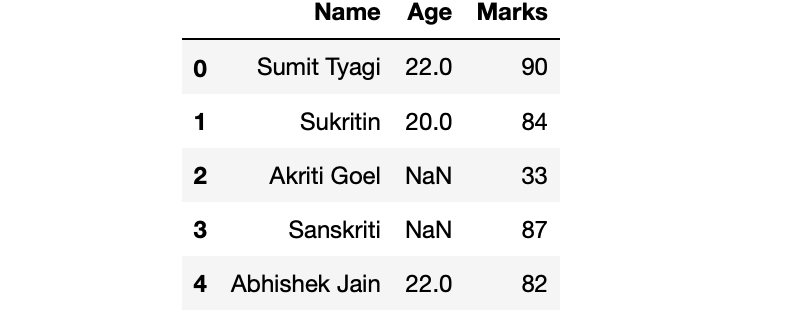Now, come to the highlighting part. Our objective is to highlight those cells which have Nan values.

Method 1: Highlighting Cell with nan values

We can do this by using the highlight_null() method of DataFrame.style property.This is a property that returns a Styler object, which has useful methods for formatting and displaying DataFrames. highlight_null() method requires one string parameter (the name of the colour with which you want to highlight the cell).

Example:

## Python3

 `# Highlighting cell with nan values ` `df.style.highlight_null(``'red'``)`

Output: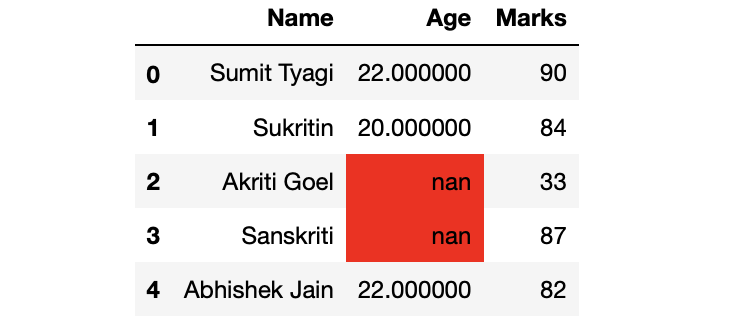Method 2: Highlighting text with nan values instead of background

We can do this by using applymap() method of the style property. applymap() method requires a function that takes a scalar and returns a scalar.
Example:

## Python3

 `# Highlighting text instead of the  ` `# cell's background ` `df.style.applymap(``lambda` `cell: ``'color:red'` `if` `pd.isnull(cell) ``else` `'')`

Output: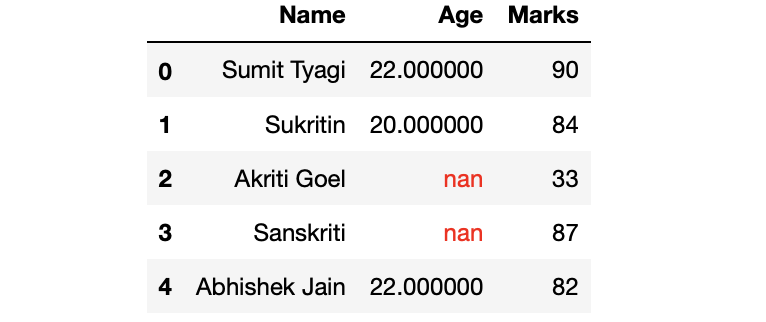Method 3: Highlighting the text of the complete row with nan values

We can do this using the apply() method
Example:

## Python3

 `# Highlighting text of the complete row ` `df.style.``apply``(``lambda` `row: np.repeat(``'color: red'` `if` `row.isnull().``any``() ``else` `'', ` `                                     ``row.shape[``0``]), axis``=``1``)`

Output: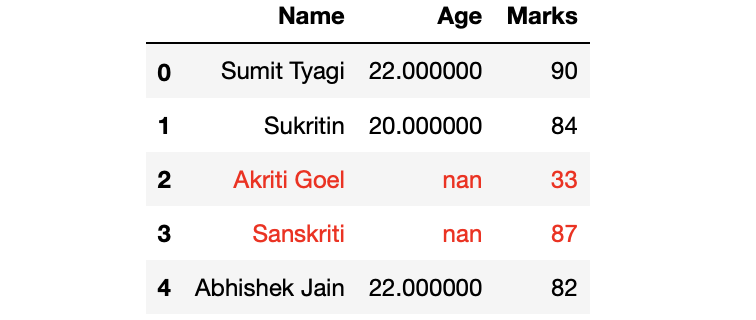Method 4: Highlighting the complete row with nan values

## Python3

 `# Highlighting the complete row ` `df.style.``apply``(``lambda` `row: np.repeat(``'background: red'` `if` `row.isnull().``any``() ``else` `'', row.shape[``0``]), axis``=``1``) `

Output: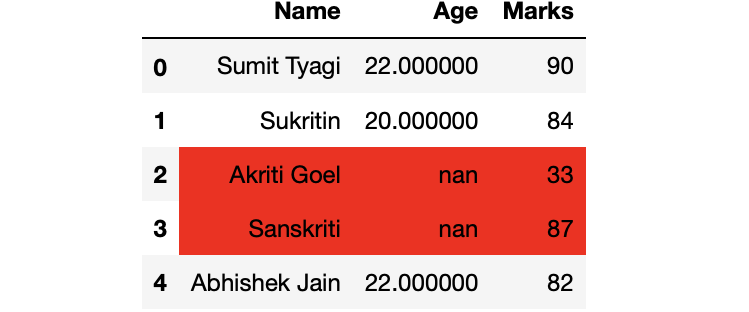## Python3

 `# Highlighting column with nan values ` `df.style.``apply``(``lambda` `row: np.repeat(``'background: red'` `if` `row.isnull().``any``() ``else` `'', ` `                                                                ``row.shape[``0``]), axis``=``0``) `

Output: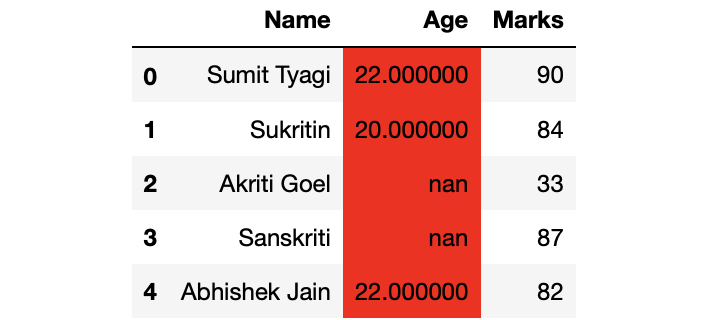Attention geek! Strengthen your foundations with the Python Programming Foundation Course and learn the basics.

To begin with, your interview preparations Enhance your Data Structures concepts with the Python DS Course.

My Personal Notes arrow_drop_up
Recommended Articles
Page :